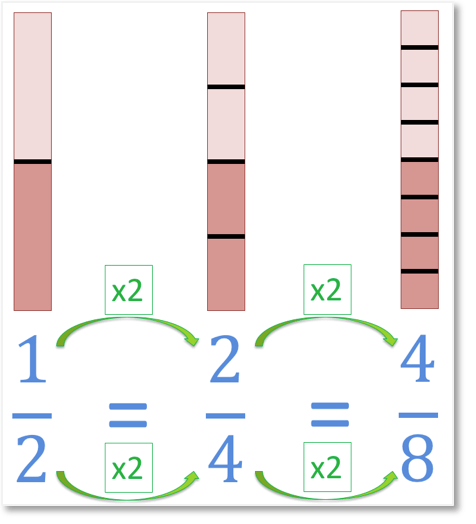# Comparing Unit Fractions

Comparing Unit Fractions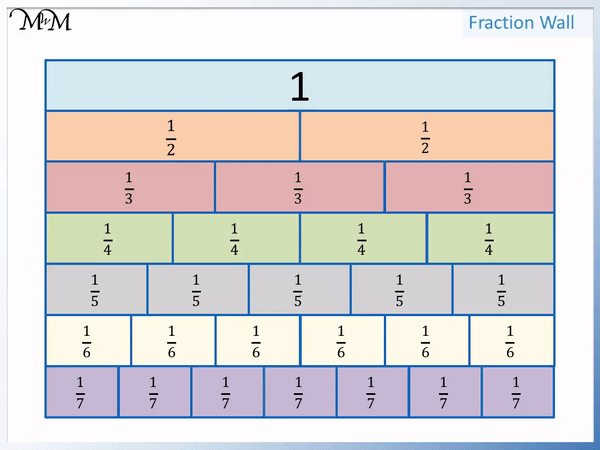• Unit fractions are fractions with a 1 as their numerator, on top of the fraction.
• The denominator is the number on the bottom of the fraction, which tells us how many parts we are dividing into.
• The larger the denominator, the more parts we divide into and the smaller the resulting fraction.
• The smaller the denominator, the fewer parts we divide into and the bigger the resulting fraction.
• We can compare the sizes of each unit fraction using a fraction wall.

Unit Fractions are fractions that have a 1 on top as their numerator.

The larger the denominator on the bottom of a unit fraction, the smaller the fraction.• Here we are comparing the unit fractions of one half and one third.
• One half means one whole divided into two parts.
• One third means one whole divided into three parts.
• We can see that one half is bigger than one third.
• The bigger the denominator on the bottom of the fraction, the more parts we have divided into.
• The bigger fraction will have a smaller denominator and the smaller fraction will have the bigger denominator.
• ‘3’ is bigger than ‘2’ and so,   1 / 2   is greater than   1 / 3  .
• The greater than sign, ‘>’ always points at the smaller fraction.#### Printable Fraction Wall# How to Compare and Order Unit Fractions

## Comparing Unit Fractions

To compare the size of two unit fractions, look at the denominators. The unit fraction with the smaller denominator is the larger fraction and the unit fraction with the larger denominator is the smaller fraction.

A unit fraction is a fraction that has a numerator of 1. The numerator is the number on top of the fraction, above the dividing line. All unit fractions have a ‘1’ on top. It does not matter what number is the denominator at the bottom of the fraction.

For example, below is an example of   1 / 4  . It is a unit fraction because it has a ‘1’ on top. It means 1 whole divided into 4 equal parts.Here is a list of some common unit fractions.A fraction wall is a common way to show the size of fractions. We will use the fraction wall to compare unit fractions.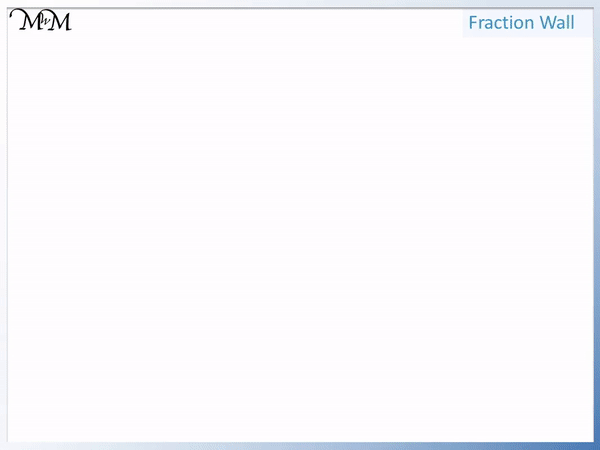We can divide one whole into two equal parts to make   1 / 2  .

We can divide one whole into three equal parts to make   1 / 3  .

We can divide one whole into four equal parts to make   1 / 4  .

We can divide one whole into five equal parts to make   1 / 5  .

We can divide one whole into six equal parts to make   1 / 6  .

We can divide one whole into seven equal parts to make   1 / 7  .We can keep dividing into smaller and smaller parts if needed.

We can compare the size of each unit fraction.

Below is a fraction wall showing the size of each unit fraction.We can see that   1 / 2  is larger than   1 / 3  , which is larger than   1 / 4  , which is larger than   1 / 5  , which is larger than   1 / 6  , which is larger than   1 / 7  and so on.We can see the size of each unit fraction below.We will look at an example of comparing two unit fractions.

In this example we have   1 / 2  compared to   1 / 3  .

The line in the fraction can be thought of as a division operation.   1 / 2  means one whole divided by 2.

1 / 3  means one whole divided by 3.We can see that one half is a bigger fraction than one third.

One third is smaller than one half because the fraction has been divided into more parts.

Dividing into a greater number of parts means that each part must be smaller because there are more of them.

As a rule the smaller the denominator, the larger the unit fraction.

We can use this rule to help compare all unit fractions. This rule only works for fractions that have a numerator of 1.

In this example we will compare 1 / 3   and 1 / 4   .

We can see that 1 / 3  is a larger fraction than 1 / 4 .This is because with 1 / 4 we have divided one whole into 4 parts, which are smaller than the parts produced when dividing into 3 parts.

Remember that the smaller the denominator, the larger the fraction. 3 is smaller than 4 and so 1 / 3 is larger than 1 / 4 .

We say that 1 / 3 is greater than 1 / 4 .

We write 1 / 3 > 1 / 4 .

> is the symbol for ‘greater than’. When using the inequality signs of < or >, the symbol can be thought of as an arrow that points to the smaller fraction.

Here is another example. We have 1 / 7 compared to 1 / 4 .

We can see that 1 / 7 is a smaller fraction than 1 / 4 .Since both fractions are unit fractions, the one with the largest denominator is the smallest fraction.

7 is larger than 4 and so, 1 / 7 is less than 1 / 4 .

We can write this as 1 / 7 < 1 / 4 .

‘<' is the 'less than' sign. Remember that these symbols always point to the smaller fraction.

The < symbol points to 1 / 7 because this fraction is smaller than 1 / 4 .

## How to Order Unit Fractions

Unit fractions can be ordered from smallest to largest by arranging them from the largest denominator to the smallest denominator.

The unit fraction with the largest denominator is the smallest and the unit fraction with the smallest denominator is the largest.

We can simply look at the denominators and write the fractions going from largest denominator to smallest.

Here is an example of ordering unit fractions.

We have the following fractions:

•   1 / 5
•   1 / 2
•   1 / 6
•   1 / 3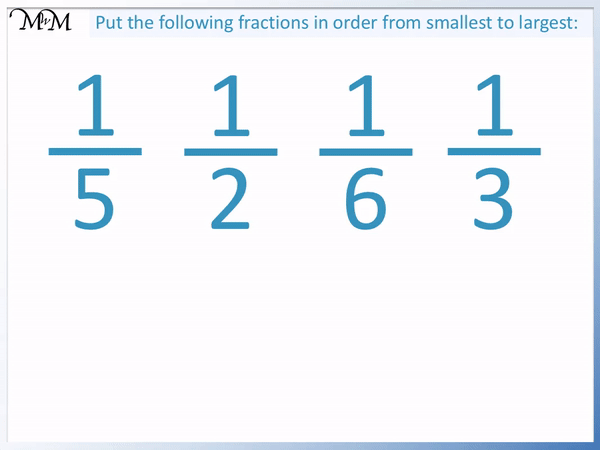Look for the largest denominator first because this is the smallest fraction.

1 / 6 contains a 6 as the denominator, which is the largest number on the bottom of these fractions. Therefore it is the smallest fraction and we write it first.

The next largest denominator is 5 and so   1 / 5 is the 2nd smallest of these units fractions.

Then we have   1 / 3 and finally,   1 / 2 .

We can write the fractions in order by simply looking at the denominators and go from largest to smallest. This is the opposite to the order than normal counting numbers usually go.

When teaching ordering unit fractions, it is possible that your child may put the fractions in the wrong order from largest to smallest because it is so natural to write numbers from smallest to largest.

Simply refer back to the visual images of the unit fractions to remind them that the larger the numerator, the smaller the fraction.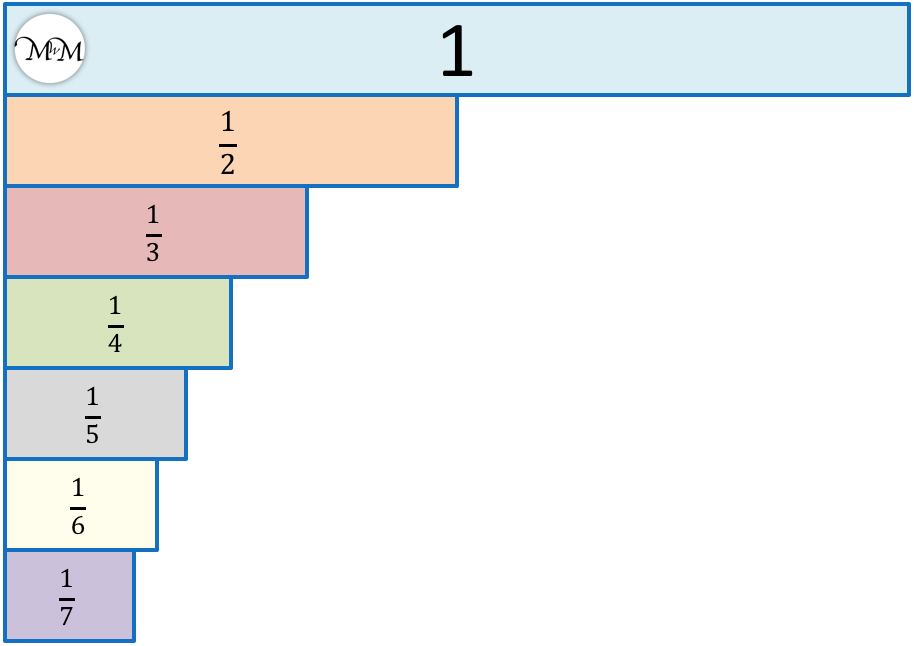Here is another example of ordering a list of unit fractions from smallest to largest.

We have:

•   1 / 8
•   1 / 3
•   1 / 4
•   1 / 10We write the unit fractions in order by going from largest denominator down to smallest.

In order we have   1 / 10 ,   1 / 8 ,   1 / 4 and finally,   1 / 3 .

1 / 10 is the smallest of these fractions and   1 / 3 is the largest of these fractions.Now try our lesson on Equivalent Fractions where we learn how different fractions can be the same size.